# Compounds Molecular and Ionic Molecular Compounds o Molecular

• Slides: 24Compounds Molecular and Ionic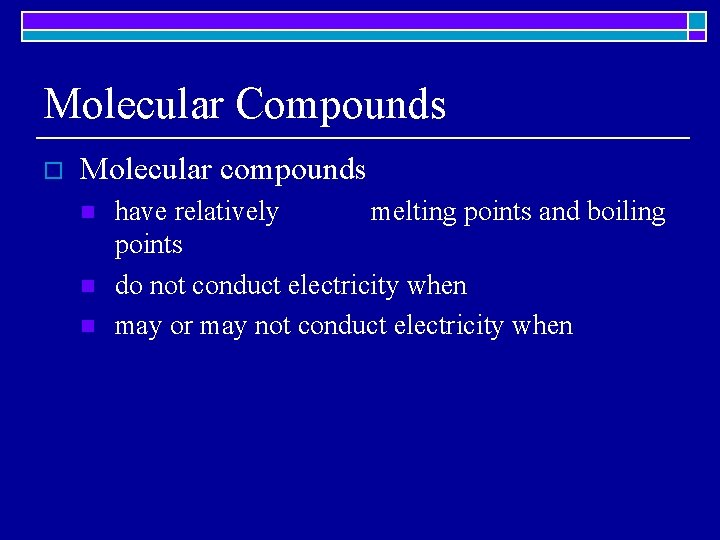Molecular Compounds o Molecular compounds n n n have relatively melting points and boiling points do not conduct electricity when may or may not conduct electricity whenMolecular Compounds o Molecular compounds n n n exist as with definite composition have individual molecules that are held together by relatively that are generally proportional to the size of the molecule for the binary compound AB, the A is attracted than to other B’sMolecular Compounds B o A Imagine that these are binary molecular compounds where the red end represents element A and the white end represents element B. A is attracted to all B’s but to one B more than the others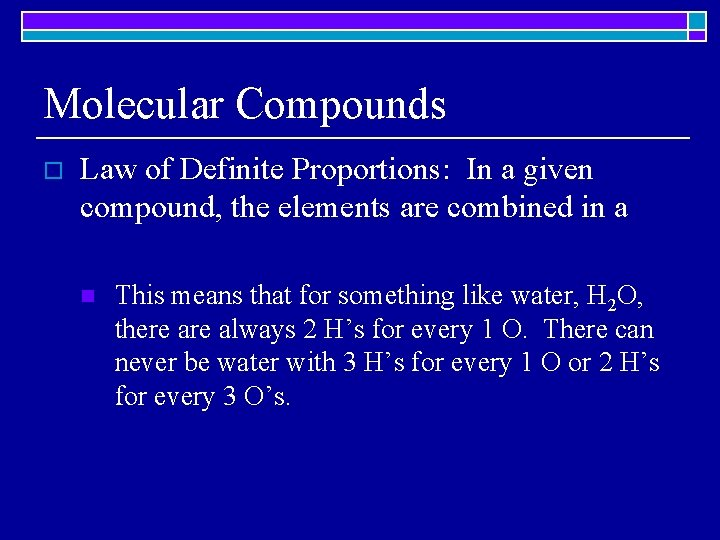Molecular Compounds o Law of Definite Proportions: In a given compound, the elements are combined in a n This means that for something like water, H 2 O, there always 2 H’s for every 1 O. There can never be water with 3 H’s for every 1 O or 2 H’s for every 3 O’s.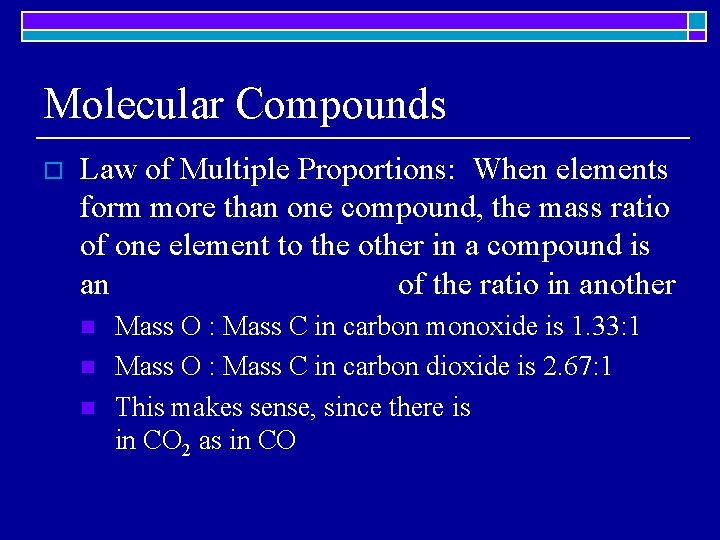Molecular Compounds o Law of Multiple Proportions: When elements form more than one compound, the mass ratio of one element to the other in a compound is an of the ratio in another n n n Mass O : Mass C in carbon monoxide is 1. 33: 1 Mass O : Mass C in carbon dioxide is 2. 67: 1 This makes sense, since there is in CO 2 as in COBinary Molecular Compounds o o A binary molecular compound is composed of two To name a binary molecular compound, it is necessary to use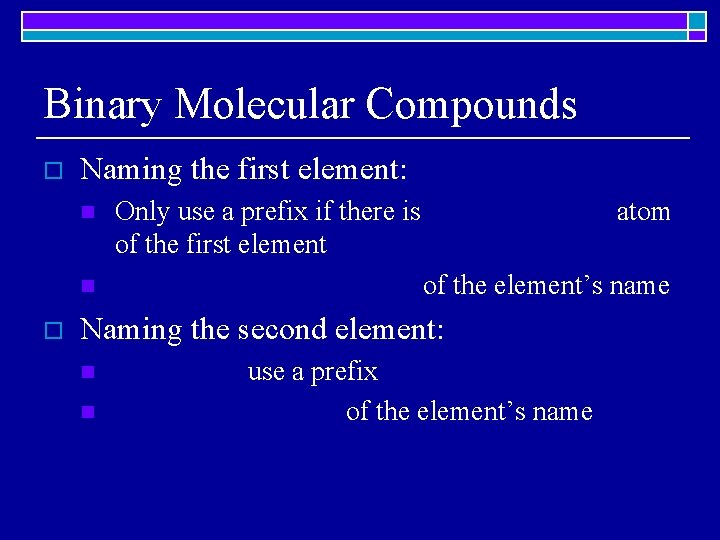Binary Molecular Compounds o Naming the first element: n n o Only use a prefix if there is of the first element atom of the element’s name Naming the second element: n n use a prefix of the element’s nameBinary Molecular Compounds o The prefixes are: n n n 1 -mono 2 -di 3 -tri n 5 -penta 6 -hexa 7 -hepta n n n 9 -nona 10 -deca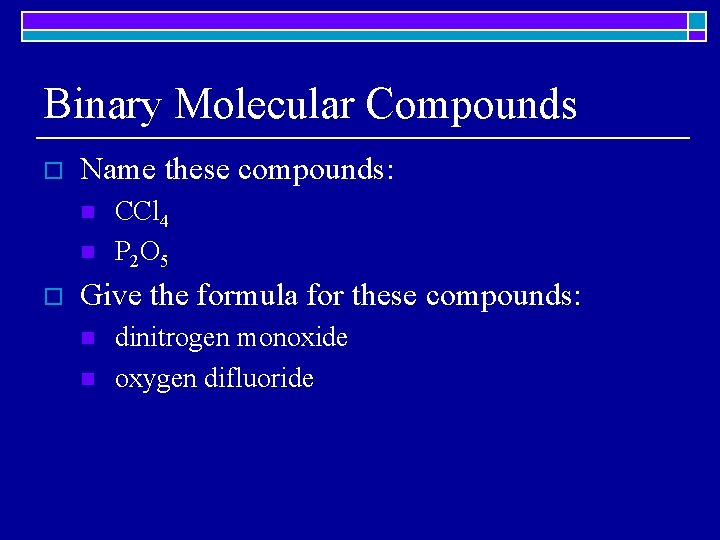Binary Molecular Compounds o Name these compounds: n n o CCl 4 P 2 O 5 Give the formula for these compounds: n n dinitrogen monoxide oxygen difluoride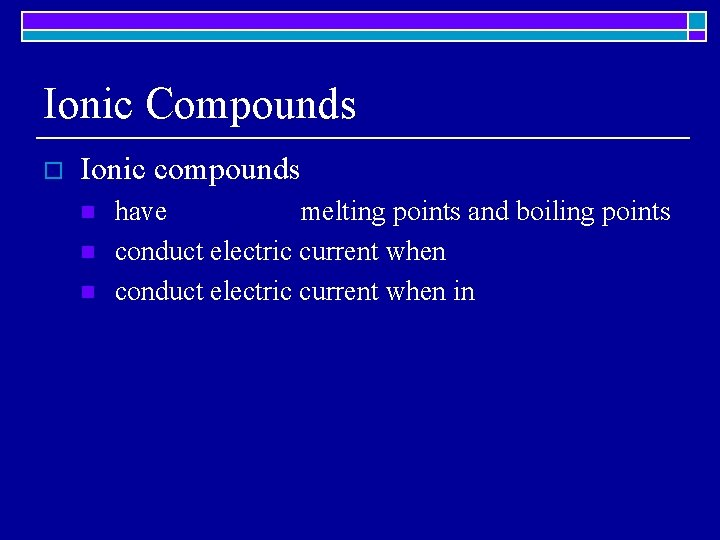Ionic Compounds o Ionic compounds n n n have melting points and boiling points conduct electric current when inIonic Compounds o Ionic compounds n n have a basic unit that is a of oppositely charged particles called have empirical formulas giving the simplest integer ratio of the ions in a o Na. Cl is not a molecule but a series of Na+ ions surrounded by Cl- ions n n Each Na+ ion is attracted equally by Each Cl- ion is attracted equally by Cl- ions Na+ ions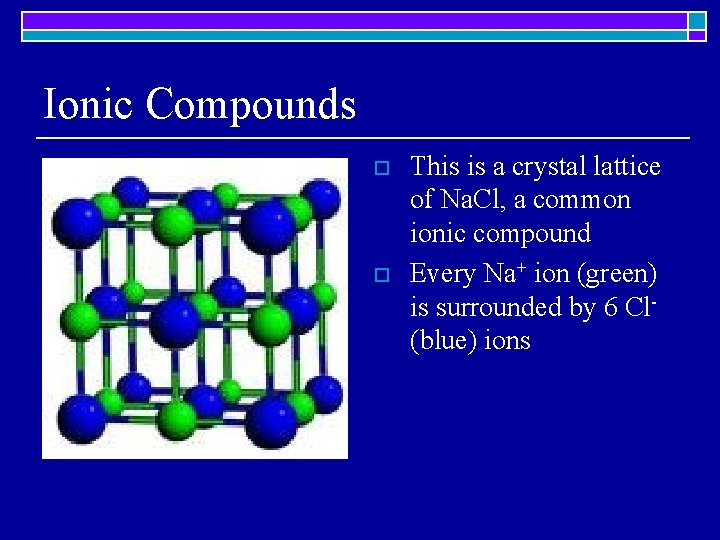Ionic Compounds o o This is a crystal lattice of Na. Cl, a common ionic compound Every Na+ ion (green) is surrounded by 6 Cl(blue) ions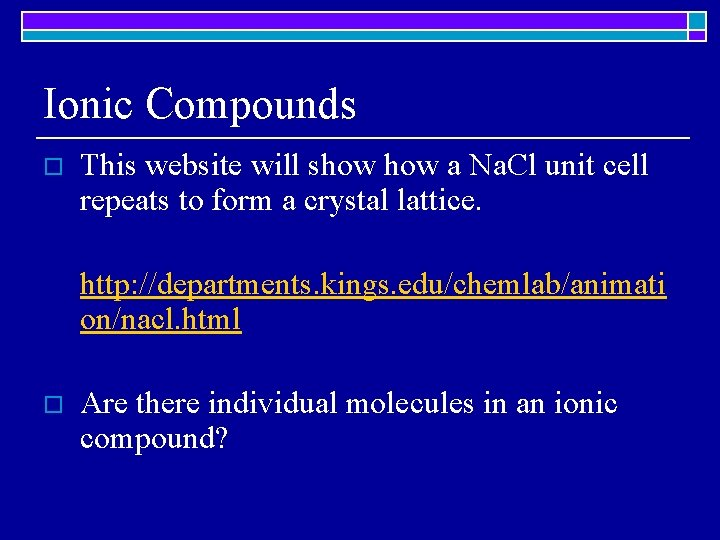Ionic Compounds o This website will show a Na. Cl unit cell repeats to form a crystal lattice. http: //departments. kings. edu/chemlab/animati on/nacl. html o Are there individual molecules in an ionic compound?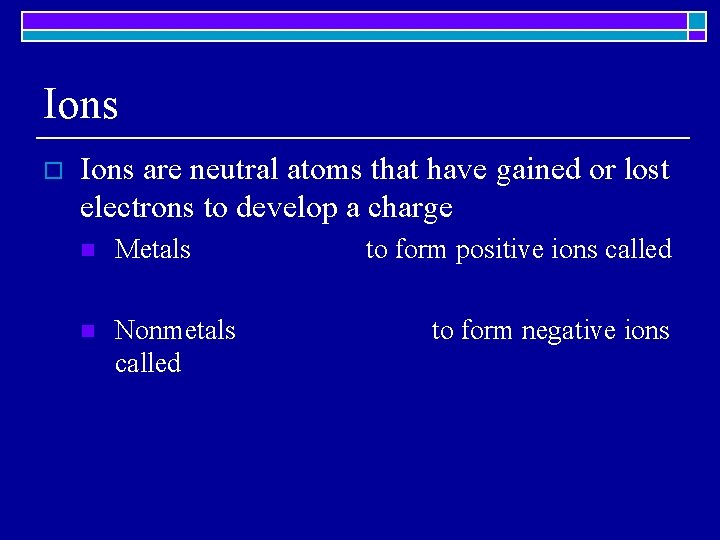Ions o Ions are neutral atoms that have gained or lost electrons to develop a charge n Metals n Nonmetals called to form positive ions called to form negative ions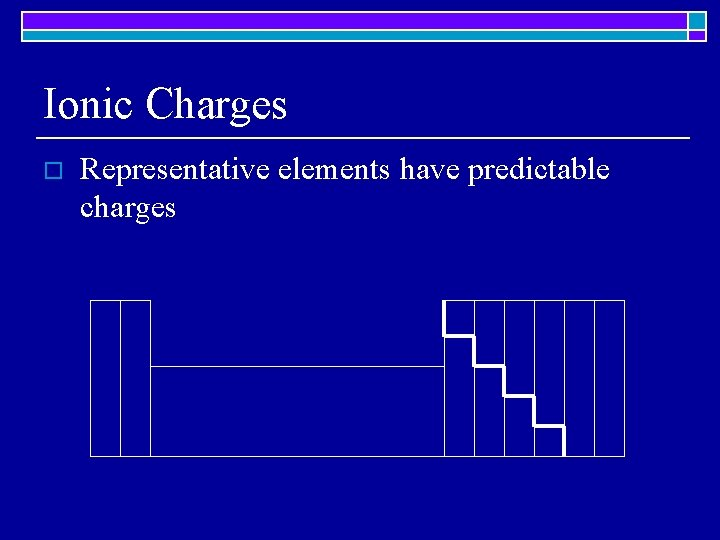Ionic Charges o Representative elements have predictable charges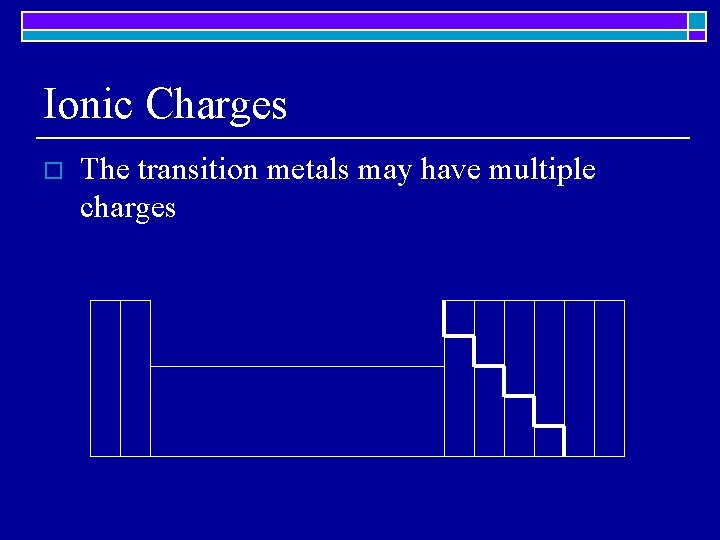Ionic Charges o The transition metals may have multiple charges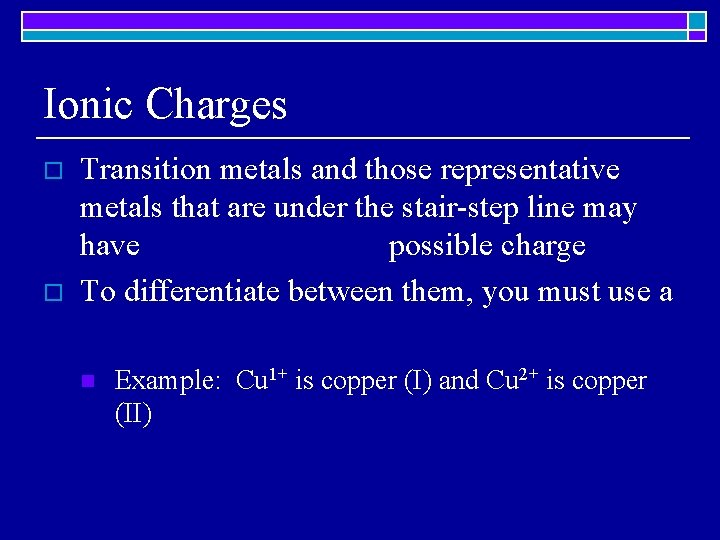Ionic Charges o o Transition metals and those representative metals that are under the stair-step line may have possible charge To differentiate between them, you must use a n Example: Cu 1+ is copper (I) and Cu 2+ is copper (II)Binary Ionic Compounds o o o Binary ionic compounds consist of ions— a cation which is a metal and an anion which is a nonmetal. Binary ionic compounds only contain The ions’ charges must cancel in order to create a formula unitBinary Ionic Compounds o Let’s say that you wanted to combine the metal Sr with the nonmetal I. n First, you would have to Sr and the charge on I o o n on Sr is in Group 2 and has a charge of I is in Group 17 and has a charge of Then, you would have to make sure the charges o It would take two I 1 -’s to cancel one Sr 2+:Naming Binary Ionic Compounds o Name the cation/metal first n NEVER use prefixees n the ending If there are multiple charges, use a n o Name the anion/nonmetal second n n NEVER use prefixes Change the ending to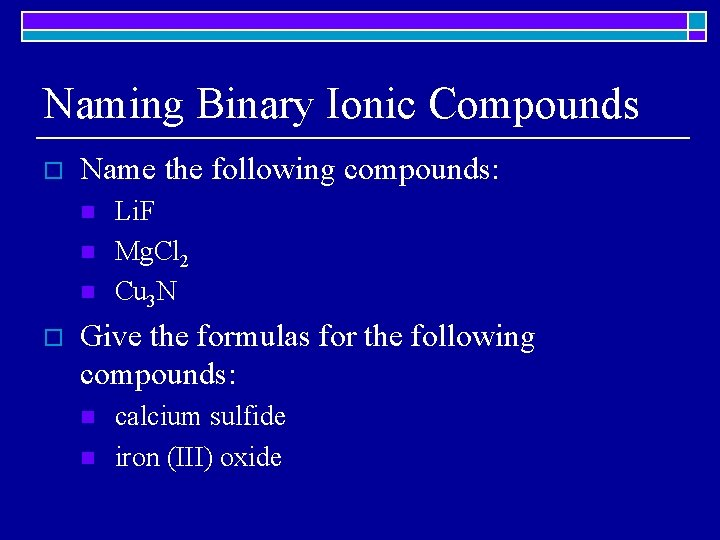Naming Binary Ionic Compounds o Name the following compounds: n n n o Li. F Mg. Cl 2 Cu 3 N Give the formulas for the following compounds: n n calcium sulfide iron (III) oxideNaming Ternary Ionic Compounds o Name the cation first n n n o Is it a polyatomic ion? If so, name it. Is it a monatomic ion? If so, check to see if it needs a and then name it. Remember, metal’s endings do not change! Name the cation second n n Is it a polyatomic ion? If so, name it. Is it a monatomic ion? If so, change the ending to.Naming Ternary Ionic Compounds o Name the following compounds n n n o Na 2 SO 4 NH 4 CO 3 Al(NO 3)3 Give the formula of the following compounds n n lead (II) acetate calcium carbonate